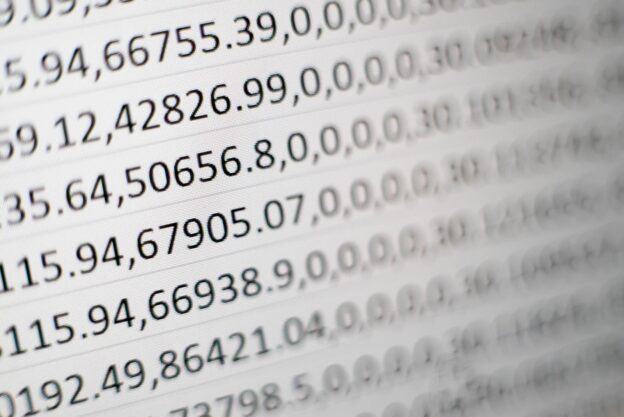# 标签归档：excel# 使用 Sheetjs 将 JSON Array 转化为 Excel

## 注意

Sheetjs 和 exceljs 不同，区分了商业版和社区版。我们这里使用的是社区版 Sheetjs CE

## 用法

``````const XLSX = require("xlsx");

const data = [
{
...
},
...
]

const worksheet = XLSX.utils.json_to_sheet(data);
const workbook = XLSX.utils.book_new();
XLSX.utils.book_append_sheet(workbook, worksheet, "sheetNameIsFirst");
XLSX.writeFile(workbook, "output.xlsx");``````# 使用 node-excel-stream 来按行处理 Excel 数据

## 读取 Excel 内容

``````let dataStream = fs.createReadStream('data.xlsx');
sheets: [{
name: 'Users',
rows: {
name: 'User Name',
}, {
name: 'Value',
key: 'value',
type: Number
}]
}
}]
})
console.log('starting parse');
reader.eachRow((rowData, rowNum, sheetSchema) => {
console.log(rowData);
})
.then(() => {
console.log('done parsing');
});``````

## 写入 Excel 内容

``````let writer = new ExcelWriter({
sheets: [{
name: 'Test Sheet',
key: 'tests',
name: 'Test Name',
key: 'name'
}, {
name: 'Test Coverage',
key: 'testValue',
default: 0
}]
}]
});
let dataPromises = inputs.map((input) => {
// 'tests' is the key of the sheet. That is used
// to add data to only the Test Sheet